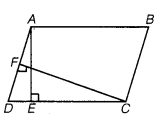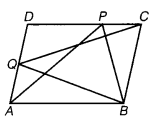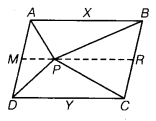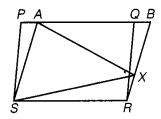# CLASS 9 MATH NCERT SOLUTION FOR CHAPTER – 9 AREAS OF PARALLELOGRAMS AND TRIANGLES EX – 9.2

## Areas of Parallelograms and Triangles

Question 1.
In the given figure, ABCD is a parallelogram, AE ⊥ DC and CF ⊥ AD. If AB =16 cm,
AE = 8 cm and CF = 10 cm, find AD.

Solution.Given, AB = 16 cm, AE = 8 cm and CF = 10 cm, D E
We know that
Area of a parallelogram = Base x Height = DC x AE
[ ∵  opposite sides of a parallelogram are equal i.e., AB = DC = 16 cm]
= 16 x 8 = 128 cm2…………….(i)
Area of a parallelogram = AD x CF
From Eqs. (i) and (ii), we have
⇒ AD =$\frac { 128 }{ 10 }$= 12.8 cm.
Hence, the value of AD is 12.8 cm.

Question 2.
If E, F, G and H are respectively the mid-points of the sides of a parallelogram ABCD, show that ar (||gm EFGH) =$\frac { 1 }{ 2 }$ ar (||gm ABCD).

Solution.Given: E, F, G, and H are respectively mid-points of the sides AB, BC, CD, and AD.
To prove : ar (||gm EFGH) =$\frac { 1 }{ 2 }$ (||gm ABCD)
Construction : Join HF.
Proof : Since, H and F are mid-points of AD and BC, respectively.
∴ DH =$\frac { 1 }{ 2 }$AD and CF =$\frac { 1 }{ 2 }$ BC ……..(i)
Now, as ABCD is a parallelogram.$\frac { 1 }{ 2 }$ AD =$\frac { 1 }{ 2 }$BC and DH || CF
⇒ DH = CF and DH || CF
So, HDCF is a parallelogram. [∵ a pair of opposite sides are equal and parallel]
Now, as the parallelogram HDCF and Δ HGF stand on the same base HF and lie between the same parallel lines DC and HF.
Similarly, ar (Δ HGF) =$\frac { 1 }{ 2 }$ ar ( ||gm  HBFH)……….(ii)
similarly, ar (Δ HGF) =$\frac { 1 }{ 2 }$ ar ( ||gm  ABFH)……….(iii)
On adding eqs. (ii) and (iii), we get
ar ( Δ HGF) + ar (Δ HEF) = i [ar (||gm HDCF) + ar (||gm ABFH)]
⇒ ar (EFGH) =$\frac { 1 }{ 2 }$ ar( ||gm ABCD)
Hence Proved

Question 3.
P and Q are any two points lying on the sides DC and AD respectively of a parallelogram ABCD. Show that ar (Δ APB) ar (Δ BQC).

Solution.Given: In parallelogram ABCD, P and Q are any two points lying on the sides DC, and AD, respectively.
To prove : ar (Δ APB) = ar (Δ BQC)
Proof : Here, parallelogram ABCD and ABQC stand on the same base BC and lie between the same parallel BC and AD.
ar(ΔBQC) =$\frac { 1 }{ 2 }$ ar (||gm ABCD)        … (i)
Similarly, Δ APB and parallelogram ABCD stand on the same base AB and lie between the same parallel AB and CD.
∴ ar(Δ APB) =$\frac { 1 }{ 2 }$ ar (||gm ABCD)   … (ii)
From eqs. (i) and (ii), we get
ar(Δ APB) = ar(Δ BQC)
Hence Proved.

Question 4.
In the figure, P is a point in the interior of a parallelogram ABCD. Show that

(i) ar (Δ APB) + ar(Δ PCD) =$\frac { 1 }{ 2 }$ ar(||gm ABCD)
(ii) ar (Δ APD) + ar (Δ APBC) = ar (Δ APB) + ar(Δ PCD).
[Hint:
Through P, draw a line parallel to AB]Solution.Given : ABCD is a parallelogram.
i.e., AB || CD and AD || BC
To prove :

(i) ar (Δ APB) + ar (Δ PCD) =$\frac { 1 }{ 2 }$ ar(||gm ABCD)
(ii) ar(Δ APD) + ar (Δ PBC) = ar (Δ APB) + ar (Δ PCD)

Proof:
(i)
Through the point P, draw MR parallel to AB.
∵ MR || AB and AM || BR [∵ AD || BC]
So, ABRM is a parallelogram.
Now, as Δ APB and parallelogram ABRM are on the same base AB and between the same parallels AB and MR.
∴ ar (Δ APB) =$\frac { 1 }{ 2 }$ ar (||gm ABRM)
ar(Δ PCD) = ar (||gm MRCD)
Now, ar (ΔAPB) + ar (ΔPCD) =$\frac { 1 }{ 2 }$ ar (IP ABRM) +$\frac { 1 }{ 2 }$ ar (||gm  MRCD)$\frac { 1 }{ 2 }$ ar (||gm  ABCD) …(i)

(ii) Clearly, ar (||gm  ABCD) = ar (ΔAPD) +ar (ΔPBC) + ar (Δ APB) + ar(Δ PCD)
= ar (ΔAPD) + ar (ΔPBC) +$\frac { 1 }{ 2 }$ ar (||gm ABCD)     [from eq. (i)]
∴ ar(Δ APD) + ar (Δ PBC) = ar (||gm  ABCD) –$\frac { 1 }{ 2 }$ ar (||gm  ABCD)
ar (||gm  ABCD)($1-\frac { 1 }{ 2 }$)
⇒ ar(Δ APD) + ar(Δ PBC) = – ar (||gm ABCD)   … (ii)
From eqs. (i) and (ii), we get
ar (Δ APD) + ar (Δ PBC) = ar (Δ APB) + ar (Δ PCD) Hence proved.

Question 5.
In the figure, PQRS and ABRS are parallelograms and X is any point on side BR. Show that

(i) ar (||9m PQRS) = ar (||gm ABRS)
(ii) ar (Δ AXS) =  ar (||gm PQRS)Solution.
Given: PQRS and ABRS both are parallelograms and X is any point on BR.
To Prove : (i) ar (||gm PQRS = ar (||gm ABRS)
(ii) ar (Δ AXS) =$\frac { 1 }{ 2 }$ ar (||gm PQRS)
Proof:
(i) Here, parallelograms PQRS and ABRS lie on the same base SR and between the same parallel lines SR and
∴ ar (||gm PQRS) = ar (||gm ABRS)   … (i)

(ii) Again, Δ AXS and parallelogram ABRS lie on the same base AS and between the same parallel lines AS and
∴  ar (Δ AXS) =$\frac { 1 }{ 2 }$ ar (||gm ABRS)      … (ii)
From eqs. (i) and (ii), we get
ar (Δ AXS) =$\frac { 1 }{ 2 }$ ar (||gm PQRS)
Hence proved.

Question 6.
A farmer was having a field in the form of a parallelogram PQRS. She took any point A on RS and joined it to points P and Q. In how many parts the fields is divided? What are the shapes of these parts? The farmer wants to sow wheat and pulses in equal portions of the field separately. How should she do it?

Solution.Given, PQRS is a parallelogram and A is any point on RS. Now, join PA and  PQ. Thus, the field will fie divided into three parts and each part is in the shape of triangle.
Since, the Δ APQ and parallelogram PQRS lie on the same base PQ and between same parallel lines PQ and SR.
ar(Δ APQ) =$\frac { 1 }{ 2 }$ ar( ||gm PQRS)    …(i)
Then, remaining
ar(Δ ASP) + ar(Δ ARQ) = ar(||gm PQRS)
Now, from Eqs. (i) and (ii), we get
ar(Δ  APQ) = ar(Δ ASP) + ar(Δ ARQ)
So, farmer has two options.
Either the farmer should sow wheat and pulses in Δ APS and Δ AQR or in ar [Δ AQP and (Δ APS and Δ AQR)] separately.